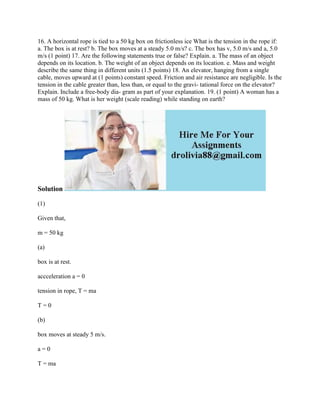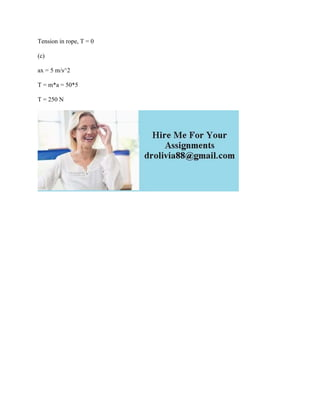Diese Präsentation wurde erfolgreich gemeldet.

# 16- A horizontal rope is tied to a 50 kg box on frictionless ice What.docx

Anzeige
Anzeige
Anzeige
Anzeige
Anzeige
Anzeige
Anzeige
Anzeige
Anzeige
Anzeige
Anzeige×

1 von 2 Anzeige

# 16- A horizontal rope is tied to a 50 kg box on frictionless ice What.docx

16. A horizontal rope is tied to a 50 kg box on frictionless ice What is the tension in the rope if: a. The box is at rest? b. The box moves at a steady 5.0 m/s? c. The box has v, 5.0 m/s and a, 5.0 m/s (1 point) 17. Are the following statements true or false? Explain. a. The mass of an object depends on its location. b. The weight of an object depends on its location. e. Mass and weight describe the same thing in different units (1.5 points) 18. An elevator, hanging from a single cable, moves upward at (1 points) constant speed. Friction and air resistance are negligible. Is the tension in the cable greater than, less than, or equal to the gravi- tational force on the elevator? Explain. Include a free-body dia- gram as part of your explanation. 19. (1 point) A woman has a mass of 50 kg. What is her weight (scale reading) while standing on earth?
Solution
(1)
Given that,
m = 50 kg
(a)
box is at rest.
accceleration a = 0
tension in rope, T = ma
T = 0
(b)
box moves at steady 5 m/s.
a = 0
T = ma
Tension in rope, T = 0
(c)
ax = 5 m/s^2
T = m*a = 50*5
T = 250 N
.

16. A horizontal rope is tied to a 50 kg box on frictionless ice What is the tension in the rope if: a. The box is at rest? b. The box moves at a steady 5.0 m/s? c. The box has v, 5.0 m/s and a, 5.0 m/s (1 point) 17. Are the following statements true or false? Explain. a. The mass of an object depends on its location. b. The weight of an object depends on its location. e. Mass and weight describe the same thing in different units (1.5 points) 18. An elevator, hanging from a single cable, moves upward at (1 points) constant speed. Friction and air resistance are negligible. Is the tension in the cable greater than, less than, or equal to the gravi- tational force on the elevator? Explain. Include a free-body dia- gram as part of your explanation. 19. (1 point) A woman has a mass of 50 kg. What is her weight (scale reading) while standing on earth?
Solution
(1)
Given that,
m = 50 kg
(a)
box is at rest.
accceleration a = 0
tension in rope, T = ma
T = 0
(b)
box moves at steady 5 m/s.
a = 0
T = ma
Tension in rope, T = 0
(c)
ax = 5 m/s^2
T = m*a = 50*5
T = 250 N
.

Anzeige
Anzeige

### 16- A horizontal rope is tied to a 50 kg box on frictionless ice What.docx

1. 1. 16. A horizontal rope is tied to a 50 kg box on frictionless ice What is the tension in the rope if: a. The box is at rest? b. The box moves at a steady 5.0 m/s? c. The box has v, 5.0 m/s and a, 5.0 m/s (1 point) 17. Are the following statements true or false? Explain. a. The mass of an object depends on its location. b. The weight of an object depends on its location. e. Mass and weight describe the same thing in different units (1.5 points) 18. An elevator, hanging from a single cable, moves upward at (1 points) constant speed. Friction and air resistance are negligible. Is the tension in the cable greater than, less than, or equal to the gravi- tational force on the elevator? Explain. Include a free-body dia- gram as part of your explanation. 19. (1 point) A woman has a mass of 50 kg. What is her weight (scale reading) while standing on earth? Solution (1) Given that, m = 50 kg (a) box is at rest. accceleration a = 0 tension in rope, T = ma T = 0 (b) box moves at steady 5 m/s. a = 0 T = ma
2. 2. Tension in rope, T = 0 (c) ax = 5 m/s^2 T = m*a = 50*5 T = 250 N